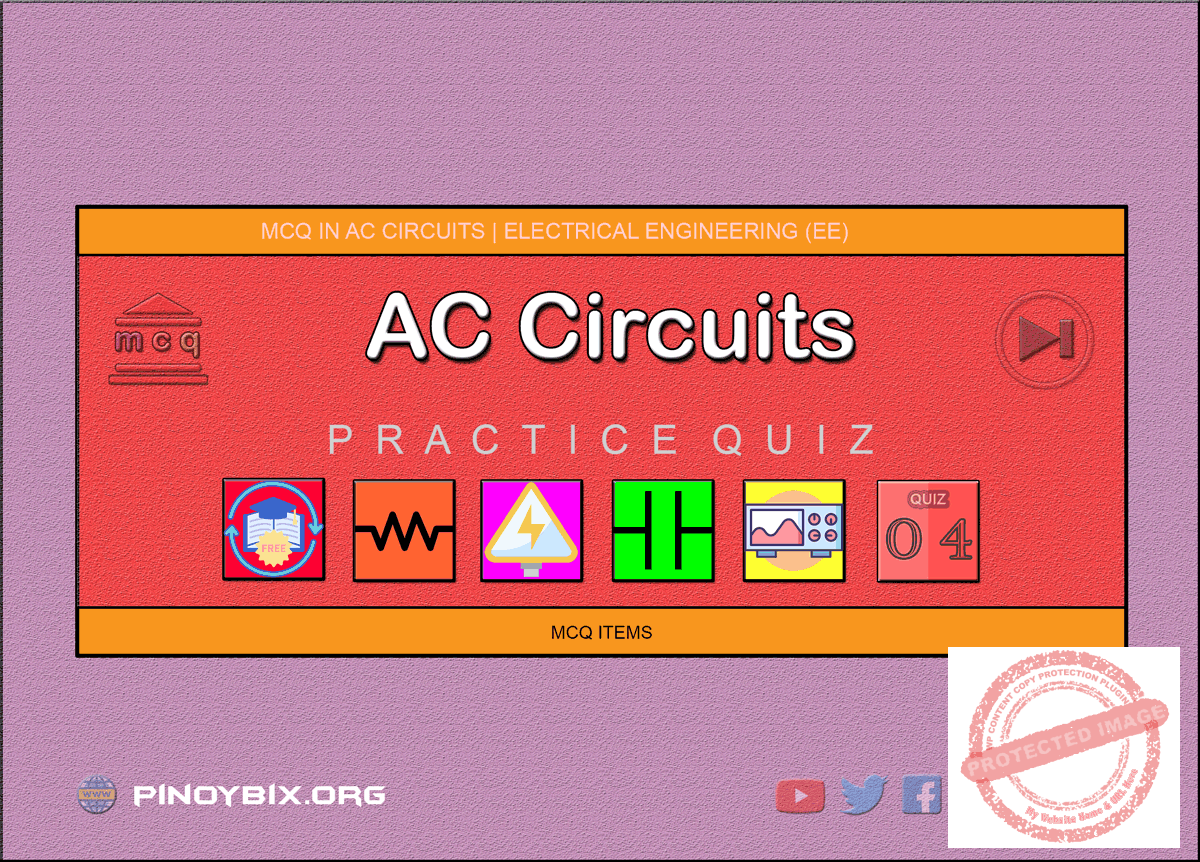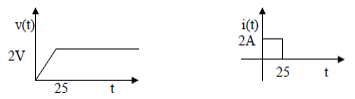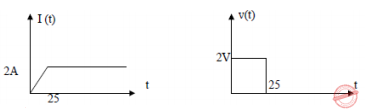# MCQ in AC Circuits Part 4 | REE Board Exam

(Last Updated On: November 13, 2020)This is the Multiple Choice Questions Part 4 of the Series in AC Circuits as one of the Electrical Engineering topic. In Preparation for the REE Board Exam make sure to expose yourself and familiarize in each and every questions compiled here taken from various sources including but not limited to past Board Exam Questions in Electrical Engineering field, Electrical Engineering Books, Journals and other Electrical Engineering References.

#### Continue Practice Exam Test Questions Part 4 of the Series

SINUSOIDAL VOLTAGE AND CURRENT

Choose the letter of the best answer in each questions.

151.  The maximum value of a sine wave AC voltage which will produce heat in a resistor at the same average rate as 115 V of direct current is ____.

A.  81.3 V

B.  115 V

C.  162.6 V

D.  230 V

Solution:

152.  A sinusoidal voltage source has a peak value of 150 volts. What equivalent DC  voltage  source  would  produce  the  same  heating  effect  in  a  1-ohm resistor?

A.  15 V

B.  212 V

C.  95 V

D.  106 V

Solution:

153.  The  effective  value  of  v(t)  =  100  +  A  sin  ωt  is  known  to  be  103.1.  The amplitude A of the sine term is ____.

A.  25

B.  4.85

C.  35.48

D.  100

Solution:

154.  An  alternating current and a direct current flow simultaneously in the same conductor. If the effective of the AC is 8 A and DC is 12 A, what will an AC ammeter read when connected in the circuit?

A.  14.42 A

B.  12 A

C.  11.66 A

D.  16.49 A

Solution:

155.  Find the reading of an AC voltmeter connected across the series source of 100 sin (ωt – π/2) and 100 sin ωt.

A.  100

B.  130.65

C.  170.71

D.  184.78

Solution:

156.  A voltage is given be v = 100 sin 314t. How long does it take this wave to complete one fourth of a cycle?

A.  20 ms

B.  10 ms

C.  5 ms

D.  1 ms

Solution:

157.  When a 15 V square wave is connected across a 50 volt AC voltmeter, it will read _____.

A.  21.21 V

B.  10.61 V

C.  15 V

D.  9.55 V

Solution:

158.  Calculate  the  effective  value of  v(t) =  100  sin  400t  + 50 sin  800t  +  10  cos 1200t V.

A.  79.5 V

B.  57.9 V

C.  112.25 V

D.  121. 52 V

Solution:

159.  The  magnetic  field  energy  of an  inductor  changes  from  maximum  value  to minimum value in 5 ms when connected to an ac source. The frequency of the source is

A.  20 Hz

B.  50 Hz

C.  200 Hz

D.  500 Hz

Solution:

160.  Non-sinusoidal waveforms are made up of

A.  different sinusoidal waveforms

B.  fundamental and even harmonics

C.  fundamental and odd harmonics

D.  even and odd harmonics only

Solution:

161.  The positive and negative halves of a complex wave are symmetrical when

A.  it contains even harmonics

B.  phase difference between even harmonics and fundamental is 0 or π

C.  it contains odd harmonics

D.  phase difference between even harmonies and fundamental is either π/2 or 3π/2

Solution:

162.  The  r.m.s.  value  of  the  complex  voltage  given  by v = 16√2 sin ωt + 12√2 sin 3ωt is

A.  20√2

B.  20

C.   28 √2

D.  192

Solution:

163.  In  a  3-phase  system,  ____th  harmonic  has  negative  phase  sequence  of RBY.

A.  9

B.  13

C.  5

D.  15

Solution:

164.  A  complex  current  wave  is  given  by  the  equation i = 14 sin ωt + 2 sin 5ωt . The r.m.s. value of the current is ____ ampere.

A.  16

B.  12

C.  10

D.  8

Solution:

165.  When pure inductive coil is fed by a complex voltage wave, its current wave

A.  has larger harmonic content

B.  is more distorted

C.  is identical with voltage wave

D.  shows less distortion

Solution:

166.  A complex voltage wave is applied across a pure capacitor. As compared to the fundamental voltage, the reactance offered by the capacitor to the third harmonic voltage would be

A.  nine times

B.  three times

C.  one-third

D.  one-ninth

Solution:

167.  Which  of  the  following  harmonic  voltage  components  in  a  3-phase  system would be in phase with each other?

A.  3rd, 9th, 15th etc.

B.  7th, 13th, 19th etc.

C.  5th, 11th, 17th etc.

D.  2nd, 4th, 6th etc.

Solution:

168.  An alternating voltage is one that

A.  varies continuously in magnitude

B.  reverses periodically in polarity

C.  never varies in magnitude

D.  both A and B

Solution:

169.  One  complete  revolution  of  a  conductor  loop  through  a  magnetic  field  is called a(n)

A.  octave

C.  cycle

D.  alternation

Solution:

170.  For a sine wave, one half cycle is often called a(n)

A.  alternation

B.  harmonic

C.  octave

D.  period

Solution:

171.  For a sine wave, the number of complete cycles per second is called the

A.  period

B.  wavelength

C.  frequency

D.  phase angle

Solution:

172.  To compare the phase angle between two waveforms, both must have

A.  the same amplitude

B.  the same frequency

C.  different frequency

D.  both A and B

Solution:

173.  The value of alternating current or voltage that has the same heating effect as a corresponding dc value is known as the

A.  peak value

B.  average value

C.  rms value

D.  peak-to-peak value

Solution:

174.  For an ac waveform, the period refers to

A.  the number of complete cycles per second

B.  the length of time required to complete one cycle

C.  the time it takes for the waveform to reach its peak value

D.  none of these

Solution:

175.  The wavelength of a radio wave is

A.  inversely proportional to its frequency

B.  directly proportional to its frequency

C.  inversely proportional to its amplitude

D.  unrelated to its frequency

Solution:

176.  Unless indicated otherwise, all sine wave ac measurements are in

A.  peak-to-peak values

B.  peak values

C.  rms values

D.  average values

Solution:

177.  A  unit  step  voltage  is  applied  across  an  inductor.  The  current  through  the inductor will be

A.  zero for all time

B.  a step function

C.  a ramp function

D.  a delta (impulse) function

Solution:

178.  A  ramp  current  flowing  through  an  initially  relaxed  capacitor  will result  in a voltage across it that

A.  varies inversely with time

B.  remains constant

C.  varies directly with time

D.  varies as the square of time

Solution:

179.  The voltage v(t) = t u(t) volts is connected across a 1 H inductor having an initial current of -1 A. The net current will be zero at time t equal to

A.  0

B.   1√2  seconds

C.  √2  seconds

D.  1 second

Solution:

180.  A voltage waveform v (t) = 12t2 is applied across 1H inductor for t ≥ 0, with initial current through it  being zero. The current through the inductor for t  ≥  0 is given by

A.  12t

B.  24t

C.  12t3

D.  4 t3

Solution:

181.  It is desired to have a constant direct current i(t) through the ideal inductor L. The nature of the voltage source v(t) must

A.  constant voltage

B.  linearly increasing voltage

C.  an ideal impulse

D.  exponentially increasing voltage

Solution:

182.  For the current and voltage waveforms, identify the element & its value.A.  L, 25 H

B.  C, 25 F

C.  L, 2  H

D.  C, 2 F

Solution:

183.  The voltage and current waveforms for an element are shown in  the  figure. Find the circuit element and its value.A.  L and 25 H

B.  C and 25 F

C.  L and 1 H

D.  C and 1 F

Solution:

184.  What  is  the  rms  value  of  a  square  wave  with  an  amplitude  of  10  A  and frequency of 1 Hz?

A.  0 A

B.  10 A

C.  5 A

D.  7.07 A

Solution:

185.  What is the frequency in kHz of a radio signal whose wavelength is 15 m?

A.  10,000

B.  20,000

C.  15,000

D.  20,500

Solution:

SERIES CIRCUITS

186.  REE Board Exam September 2003

The following are in series R = 1,000 Ω, L = .100 μH and C = 20,000 pF. The voltage  across  the  circuit  is  100  V,  60  kHz.  What  is  the  total  impedance expressed in ohms?

A.  1882 ohms

B.  1000 ohms

C.  2132 ohms

D.  1885 ohms

Solution:

187.  REE Board Exam October 2000

A series circuit has an applied voltage of v = 220 sin (ωt + 30°) and draws a current of i = 10 sin (ωt – 30°). What is the average power and power factor of the circuit?

A.  1,905 W, 86.6% lagging

B.  1,905 W, 86.6% lagging

C.  2,200 W, 100%

D.  1,100 W, 50% lagging

Solution:

188.  REE Board Exam September 2001

A coil has an impedance of 75.4 Ω when connected a across a source of 60 Hz. The same coil yields an impedance of 54.8 Ω when connected across a source having a different frequency of 30 Hz. What is the coil’s inductance?

A.  245.7 mH

B.  512.8 mH

C.  158.6 mH

D.  341.7 mH

Solution:

189.  REE Board Exam April 1996

A circuit consists of a 4 ohms resistor and a 300 μF capacitor in series. It is connected across a 60 Hz voltage source with a 500 V peak voltage. What is the phasor form of the current?

A.  I = 19.57∠57.5° A

B.  I = 8.84∠73.2° A

C.  I = 36.5∠65.7° A

D.  I = 10.5∠65.7° A

Solution:

190.  REE Board Exam September 2000

Find the power in a circuit if i(t) = 10 sin (ωt  –  30°) and v(t) = 220 sin (ωt +30°).

A.  550 watts

B.  2200 watts

C.  1900 watts

D.  1500 watts

Solution:

191.  REE Board Exam April 1997

A current of 2.5 A flows through a series circuit consisting of a 100 Ω resistor and  an  unknown  capacitor  across  a  source  of  460  V,  50  Hz.  What  is  the value of the capacitive reactance?

A.  XC = 91.86 Ω

B.  XC = 39.19 Ω

C.  XC = 154.45 Ω

D.  XC = 184.0 Ω

Solution:

192.  REE Board Exam April 1995

In a series RC circuit the voltage across the  capacitor and the resistor are 60 volts and 80 volts respectively. The total voltage is

A.  70

B.  100

C.  140

D.  none of these

Solution:

193.  EE Board Exam October 1984

An industrial coil has a resistance of 32 ohms and reactance of 24 ohms and rated 440  volts  at  60  Hz.  A  factory  will connect  the  coil to  a  440  V,  50  Hz supply. Solve for the value of a series resistor needed to avoid over-current condition.

A.  2.07 ohms

B.  2.64 ohms

C.  2.44 ohms

D.  2.25 ohms

Solution:

194.  REE Board Exam October 1998

Two  relays  each  with  20  ohms  resistance  and  0.16  H  inductance  are connected in series. What is the equivalent impedance?

A.  20 + j102.2 Ω

B.  20 + j95.32 Ω

C.  40 + j120.63 Ω

D.  40 + j25.32 Ω

Solution:

195.  EE Board Exam October 1990

An  inductive  coil  takes  a  current  of  2  A  and  consumes  160  W  when connected to a 240 V ac supply. A second coil when connected across the same supply takes 3 A and 500 W. Find the total power when the two coils are connected in series to this supply,

A.  144.56 W

B.  134.31 W

C.  150.22 W

D.  128.35 W

Solution:

196.  EE Board Exam October 1985

A coil draws 1875 watts when connected to a 150 V dc source. It consumes 30.72 watts when use on a 240 V, 60 Hz ac source. Find the inductance of the coil.

A.  0.0255 H

B.  0.0341 H

C.  0.0153 H

D.  0.0240 H

Solution:

197.  REE Board Exam October 1994

A  current  of  10  A  and  a power  factor  of  0.8  lagging  is  taken  form  a  single phase 250 volt supply. The reactive power of the system is

A.  1500 vars

B.  2000 vars

C.  2500 vars

D.  none of these

Solution:

198.  REE Board Exam October 1996

The resistor of 6 Ω and unknown impedance coil in series draws 12 A from a 120 V, 60 Hz line. If the real power taken from the line is 1152 watts, what is the coil inductance?

A.  15.9 mH

B.  10 mH

C.  20 mH

D.  1.59 mH

Solution:

199.  REE Board Exam April 1997

Determine the power factor angle in the series circuit which consists of R = 25 Ω, L = 0.2 H, across a power supply of 200 V, 30 Hz.

A.  36.4°

B.  46.4°

C.  52.4°

D.  56.4°

Solution:

200.  EE Board Exam April 1993

The impedance coils absorbs 250 watts when  connected across 220 V, 60 Hz mains. It is then connected across 110 V, 25 Hz mains and also absorbs 250 watts. What is the inductance of the coil?

A.  0.125 H

B.  0.149 H

C.  0.154 H

D.  0.163 H

Solution:

#### Questions and Answers in AC Circuits

Following is the list of practice exam test questions in this brand new series:

MCQ in AC Circuits
PART 1: MCQ from Number 1 – 50                  Answer key: included
PART 2: MCQ from Number 51 – 100              Answer key: included
PART 3: MCQ from Number 101 – 150            Answer key: included
PART 4: MCQ from Number 151 – 200            Answer key: included
PART 5: MCQ from Number 201 – 250             Answer key: included
PART 6: MCQ from Number 251 – 300             Answer key: included
PART 7: MCQ from Number 301 – 350             Answer key: included
PART 8: MCQ from Number 351 – 400             Answer key: included
PART 9: MCQ from Number 401 – 450             Answer key: included
PART 10: MCQ from Number 451 – 500           Answer key: included

P inoyBIX educates thousands of reviewers and students a day in preparation for their board examinations. Also provides professionals with materials for their lectures and practice exams. Help me go forward with the same spirit.

“Will you subscribe today via YOUTUBE?”

Subscribe

PinoyBIX Engineering. © 2014-2020 All Rights Reserved | How to Donate? |